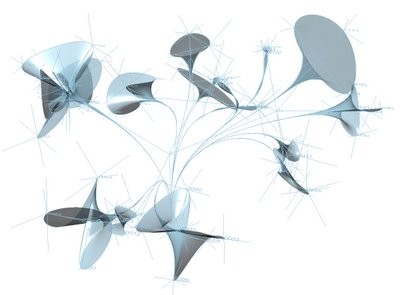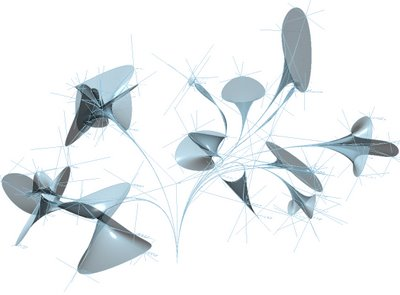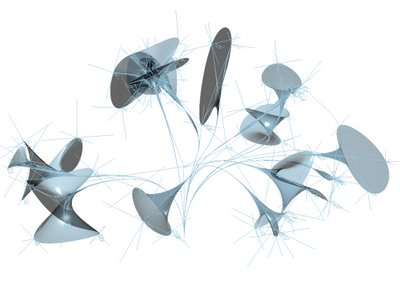## Tuesday, August 01, 2006

### Rh4_060801_onTheWayTo_L-SytemsFROM FRACTALS TO L-SYSTEMS...
going from unifrom growing generation to an alphabet based one...

strCase = Fix(random(2,4))
Select Case CStr(strCase)
Case 2 ...
Case 3 ...
Case 4 ...
End Select

L-SYSTEMS

"The recursive nature of the L-system rules leads to self-similarity and thereby fractal-like forms which are easy to describe with an L-system. Plant models and naturally-looking organic forms are similarly easy to define, as by increasing the recursion level the form slowly 'grows' and becomes more complex.

L-system grammars are very similar to the semi-Thue grammar (see Chomsky hierarchy). L-systems are now commonly known as parametric L systems, defined as a tuple

G = {V, S, ω, P},

where

* V (the alphabet) is a set of symbols containing elements that can be replaced (variables)
* S is a set of symbols containing elements that remain fixed (constants)
* ω (start, axiom or initiator) is a string of symbols from V defining the initial state of the system
* P is a set of rules or productions defining the way variables can be replaced with combinations of constants and other variables. A production consists of two strings - the predecessor and the successor.

The rules of the L-system grammar are applied iteratively starting from the initial state.

An L-system is context-free if each production rule refers only to an individual symbol and not to its neighbours. If a rule depends not only on a single symbol but also on its neighbours, it is termed a context-sensitive L-system.

If there is exactly one production for each symbol, then the L-system is said to be deterministic (a deterministic context-free L-system is popularly called a D0L-system). If there are several, and each is chosen with a certain probability during each iteration, then it is a stochastic L-system.

i.e. Wikipedia